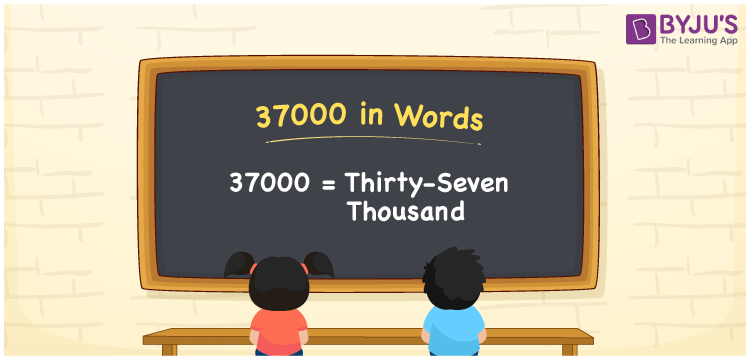# 37000 in words

Thirty-seven thousand can be written as 37000 in words. If you purchased a new gadget for Rs. 37000, you may remark, “I purchased a new gadget worth Thirty-seven thousand rupees.” In this post, you’ll discover a fun way to translate the number 37000 into English.

 37000 in words Thirty-seven Thousand Thirty-seven Thousand in Numbers 37000

## 37000 in English Words

We generally write numbers in words using the English alphabet. Thus, we can read 37000 in English as “Thirty-seven Thousand”.## How to Write 37000 in Words?

In this section, you will learn how to write the number 37000 in words. The given number 37000 has five digits, so we should make a place value chart that represents the place value for all these five digits. This can be done as follows.

 Ten thousands Thousands Hundreds Tens Ones 3 7 0 0 0

This can be written as:

3 × Ten thousand + 7 × Thousand + 0 × Hundred + 0 × Ten + 0 × One

= 1 × 30000 + 7 × 1000  + 0 × 100 + 0 × 10 + 0 × 1

= 30000 + 7000

= 37000

= Thirty-seven Thousand

Thus, 37000 in words is written as Thirty-seven Thousand.

37000 is a natural number that precedes 37001 and succeeds 36999.

37000 in words – Thirty-seven Thousand

Is 37000 an odd number? – No

Is 37000 an even number? – Yes

Is 37000 a perfect square number? – No

Is 37000 a perfect cube number? – No

Is 37000 a prime number? – No

Is 37000 a composite number? – Yes

## Frequently Asked Questions on 37000 in Words

Q1

### How do you write 37000 in English?

We can write 37000 in English words as “Thirty-seven Thousand”.
Q2

### How do you write 37000 in words for a cheque?

For a cheque, we generally write 37000 in words as Thirty-seven Thousand rupees only.
Q3

### What number is Thirty-seven Thousand in words?

The number 37000 is Thirty-seven Thousand in words.